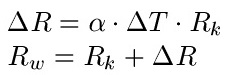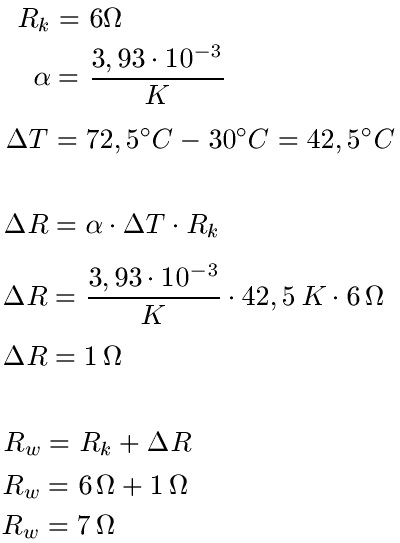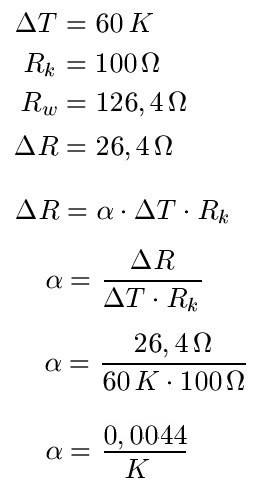# What is the temperature coefficient of resistance

## Temperature resistances / temperature dependent

Resistances depend on the temperature. But how can you calculate this temperature dependence of resistances? This is exactly what I'll show you in the next few sections. Equations and formulas are discussed and examples are calculated. This article belongs to our field of physics or electrical engineering.If electrical circuits are operated, they become warmer. This changes the resistance of cables and components. In some cases this doesn't matter at all, in others the change in the resistance values ​​is very critical. It often takes a lot of experience as a developer to evaluate this correctly. But I don't want to go that far here. The next few sections first look at how the resistance changes when the temperature changes.

There are numerous representations for equations or formulas relating to the temperature dependence of resistances. I have chosen what I consider to be the easiest representation in this article. Let's look at the two equations, then we'll discuss examples:The following applies:

• Delta R is the change in resistance in ohms
• Alpha is the temperature coefficient and depends on the material
• Delta T is the change in temperature
• R.K is the resistance value before the temperature increase
• R.W. is the resistance value after the temperature increase

Hints:

• A change in temperature of 1 degree Celsius corresponds to a change in temperature of 1 Kelvin.
• For tasks we first calculate the delta R, i.e. how much the temperature changes, and insert this into the second equation
Show:

### Calculate change in resistance Examples

Let's look at examples for a better understanding. These should clarify the use of the equations and also show how to use the units.

Example 1:

A wire made of copper has a resistance of 6 ohms at a temperature of 30 degrees Celsius. The wire is heated to 72.5 degrees Celsius. The temperature coefficient is 3.93 · 10-3 K-1. How big is the wire resistance after the temperature increase?

Solution: From the task we can see that the initial resistance - i.e. the resistance when it is even colder - with Rk = 6 ohms. The temperature coefficient alpha is also included in the exercise. However, in order to be able to start calculating, we still need Delta T. This is 42.5 degrees Celsius, because the wire is heated to this temperature. A temperature change of one degree Celsius corresponds to a temperature change of 1 Kelvin. With that we go into the first equation and calculate that the resistance value increases by 1 ohm. On top of the 6 ohm output resistance before heating, there is another 1 ohm.Example 2:

A wire is heated from 30 degrees Celsius to 90 degrees Celsius. As a result, the resistance has increased by 26.4 percent. What is the temperature coefficient of the material?

Solution: From 30 degrees Celsius to 90 degrees Celsius corresponds to a change of 60 degrees Celsius or 60 Kelvin. So we have our Delta T. But then it gets harder because we can't just plug it into one of the equations. We help ourselves differently: The resistance has increased by 26.4 percent due to the warming. A resistor would have grown from 100 ohms to 126.4 ohms. Let's just use this and use it exactly like that. And the Delta R is 26.4 ohms. We convert our equation to alpha, insert and thus get the solution.Left:

### Who's Online

We have 1108 guests online